# Electronics and Communication Engineering - Exam Questions Papers

26.

The following circuit represents :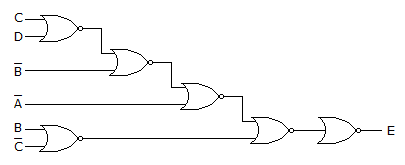A. A(B + CD) BC B.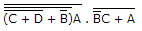C. AB + BC + ACD D. none of these

Explanation: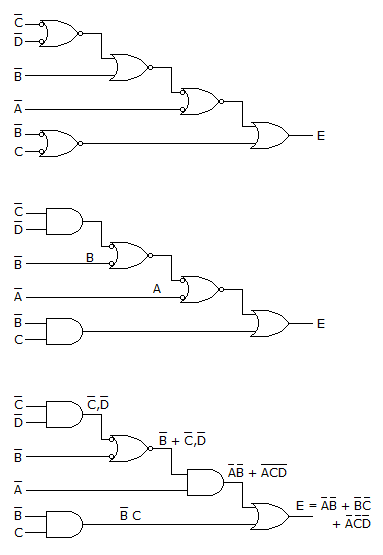27.

In the circuit shown below current through the resistor is shown. Voltage across 4 Ω resistor is: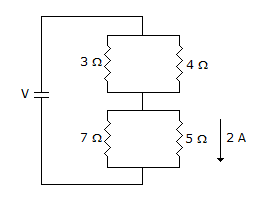A. 8 volt B. ≈ 5.87 V C. ≈ 6.87 V D. None of these

Explanation:

Voltage across 5 Ω = 10 V

Thus voltage across 7 Ω = 10 V (5 Ω || 7 Ω)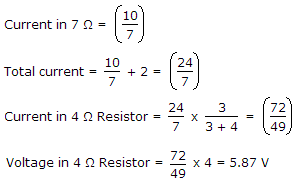28.

If the function A, B are A = R + PQ + RS B = PQRS + PQRS + PQRS
Which of the following is true?

 A. A = B B. A = B C. no direct relation between A and B D. insufficient data

Explanation:

A = R + PQ + RS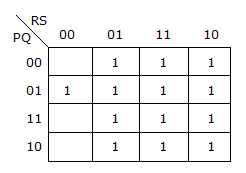B = PQ R S + P Q R S + PQ R S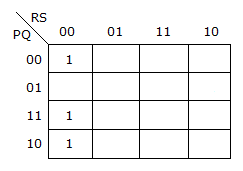29.

In 8085 microprocessor system, what will be the total delay provided by the following loop.`MVI A, 11HLXI B, 12FFHDelay: DCX BXTHLNOPXRA BJNZ Delay`
where system clock is operating at 3 MHz.

 A. 3.154 msec B. 3.514 msec C. 2.644 msec D. 3.166 msec

Explanation:

8085 T-States

` `

``` MVI A, 11H 7 LX1 B, 12FFH 10 Delay: DCX B 6 XTHL 16 NOP 4 XRA B 4 JNZ Delay 10/7 ```

Total T states = 7 + 10 + 255(6 + 16 + 4 + 4 + 7) + 10 = 9462

Therefore total delay introduced =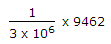= 3.154 m sec.

30.

A speech signal, band limited to 4kHz and peak voltage varying between + 5V and - 5V is sampled at the Nyquist rate. Each sample is quantized and represented by 8 bits. The number of quantization levels required to reduce the quantization noise by a factor of 4 would be

 A. 1024 B. 512 C. 256 D. 64

Explanation:

To reduce quantization noise by 4

No. of levels should increase by 2

Thus 512 levels are required.

#### Current Affairs 2022

Interview Questions and Answers